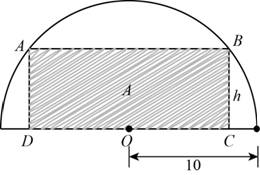# The function that models area ( A ) of a rectangle with height ( h ) inscribed in a semicircle of radius 10.### Precalculus: Mathematics for Calcu...

6th Edition
Stewart + 5 others
Publisher: Cengage Learning
ISBN: 9780840068071### Precalculus: Mathematics for Calcu...

6th Edition
Stewart + 5 others
Publisher: Cengage Learning
ISBN: 9780840068071

#### Solutions

Chapter 2, Problem 17P
To determine

## To find: The function that models area (A) of a rectangle with height (h) inscribed in a semicircle of radius 10.

Expert Solution

The model that function area of rectangle in terms of its height is A(h)=2h100h2 .

### Explanation of Solution

Given:

The given figure is,Figure (1)

Here ABCD is rectangle with height h and O is centre of semicircle with radius 10.

Calculation:

Join the points O and B in the given Figure (1) with a line to form a triangle OCB as shown in Figure (2) below.Figure (2)

In triangle OBC , OC is x , OB is radius of semicircle i.e. 10 units.

Apply Pythagorean theorem in triangle OBC and calculate x in terms of h.

(AB)2=(OC)2+(CB)2

Substitute 10 for OB , x for OC and h for CB in above equation.

(10)2=(x)2+(h)2100=x2+h2

Add h2 to both the sides of above equation.

100=x2+h2100h2=x2+h2h2100h2=x2

Take square root of above equation.

100h2=x

The formula to calculate area of rectangle is,

A=lengthwidth

Substitute 2x for length and h for width in above equation.

A=2xh

Substitute 100h2  for x in above equation.

A=2100h2hA=2h100h2

The model that function area of rectangle in terms of its height is A(h)=2h100h2 here h varies between 0<h<100 .

### Have a homework question?

Subscribe to bartleby learn! Ask subject matter experts 30 homework questions each month. Plus, you’ll have access to millions of step-by-step textbook answers!